Next: Conclusions Up: Declarative Formalization of Strategies Previous: Proof

The nested abnormality theory described bynot only solves the problem of projecting a specific strategy, it also describes a particular use of circumscription that allows reasoning about declarative formalizations of strategies of the sort proposed in this paper. The expression(strategy) denotes the nested abnormality theory described byparameterized for different strategy descriptions. The idea is to replace axiomstoby other sets of axioms describing different strategies, so that we can reason about the behavior of different strategies.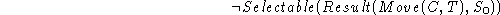We describe a scenario in which a program uses(strategy) to reason about declarative formalizations of strategies. The program starts with an empty strategy. As different heuristics are suggested by the adviser, the program considers how they may affect its problem solving behavior, and reacts accordingly.

The scenario tries to illustrate the idea that a program capable of reasoning non-monotonically about declarative formalizations of strategies can have interesting reflective behavior [McCarthy1990b] [McCarthy1995] [Steels1996] [Sierra1996]. For example, it can save computational resources by detecting uncomputable or incorrect strategies. It can determine which of the heuristics is told improve, are redundant, partially redundant, or inconsistent with its current strategy. It can improve its problem solving strategy, accordingly, by adding and substituting action selection rules and axioms. It can avoid inconsistencies, which may cause it to have an arbitrary behavior. It can learn by taking advice [McCarthy1959], and reflecting on it.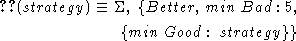Figure: Behavior of different strategies for solving planning problems in the blocks world: (1) Strategy-1 and Strategy-3 are both uncomputable, since they allow cyclic behaviors; (2) Strategy-2 describes a computable but incorrect strategy, its unique terminal situation is not a solution; (3) Strategy-4 and Strategy-5 describe two computable and correct strategies, together with their projections for a particular blocks world problem. It can be easily observed that Strategy-5 is better than Strategy-4.

Initially, the advisor suggests to use the following heuristic: If a block can be moved to final position, this should be done right away. The program constructs Strategy-1, which is described by axiom. The projection of Strategy-1 allows cyclic behaviors, such as the one described in fig.. The program concludes that Strategy-1 is not computable.

We use the following formula to identify cycles in the projections of state-based strategies. The expression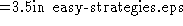means that there is a nonempty sequence of selectable situations that leads from s to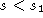. A state-based strategy contains a cycle (axiom) if there is a nonempty sequence of selectable situations that leads from a situation s to a different situationwith the same associated state (i.e., whose associated database contains the same formulas as the database associated with s).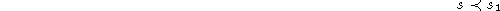In particular, the program can prove that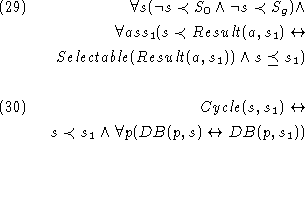holds in the projection of Strategy-1 by finding appropriate values for s and.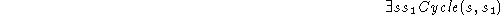The program asks for more advice, instead of trying to apply Strategy-1 to solve the problem. The advisor proposes a different heuristic: If a block is not in final position and cannot be moved to final position, it is better to move it to the table than anywhere else. The program constructs Strategy-2, which is described by axiom. The projection of Strategy-2 is shown in fig.. Strategy-2 is an incorrect strategy, because it allows terminal situations which do not satisfy the goal conditions.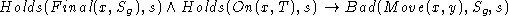The program still needs more advice. The advisor suggests now to consider both heuristics together. The program constructs Strategy-3, which is described by axiomsand. The projection of Strategy-3 still allows cyclic behaviors, such as the one described in fig., i.e. it satisfies.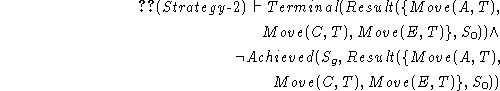The advisor suggests a third heuristic: If a block is in final position, do not move it. The program constructs Strategy-4 as the set of axiomsto. The set of situations that are selectable according to projection of Strategy-4 (see fig.) is finite. The program knows the strategy is correct, since all its terminal situations happen to be solutions. The second order axiom of induction for situations () is needed here in order to prove that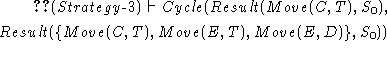holds for all the situations not mentioned in the theorem below.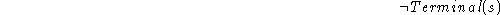The advisor suggests a fourth heuristic: If there is a block that is above a block it ought to be above in the goal configuration but it is not in final position (tower-deadlock), put it on the table. The program constructs Strategy-5 as the set of axiomsto. The set of situations that are selectable according to projection of Strategy-5 (see fig.) is finite. The program can prove that Strategy-5 is correct. It can also conclude that Strategy-5 is better than Strategy-4, since it always solves the problem performing a smaller or equal number of actions.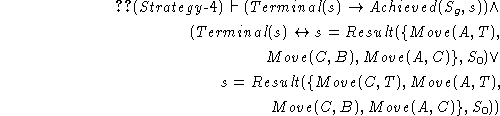The advisor still suggests a fifth heuristic: If a block is on the table but not in final position, do not move anything on that block.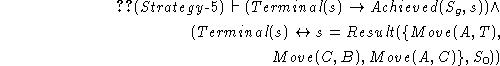The program constructs Strategy-6 as the set of axiomstoand. It can check that the projections of Strategy-5 and Strategy-6 are identical. This means that suggestionis redundant with its current strategy. Therefore, including it in the database will not improve the program's behavior.

The advisor finally suggests a sixth heuristic: If there is a block that is above a block it ought to be above in the goal configuration but it is not in final position (tower-deadlock), it is better to move it on top of a clear block that is in final position and should be clear on the goal configuration than anywhere else.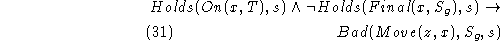We use the following formula to detect inconsistencies in the projection of a strategy. The formulas,andare examples of verification axioms that can be added to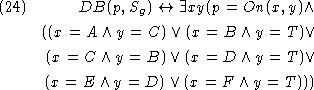in order to reason about the behavior of different strategies.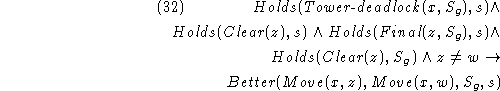The program constructs Strategy-7 as the set of axiomstoand. Although axiomdescribes a plausible heuristic, it is in contradiction with axiom. For example, axiomimplies that Move(A,T) is good in the initial situation, whereas axiomsandimply that Move(A,T) is bad. Using axiom, the program can prove that Strategy-7 is inconsistent.Therefore, it rejects suggestion, because it is inconsistent with its current strategy.Next: Conclusions Up: Declarative Formalization of Strategies Previous: Proof

Josefina Sierra
Tue Jul 21 09:54:27 PDT 1998